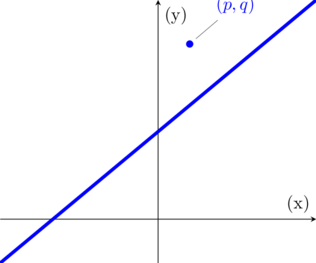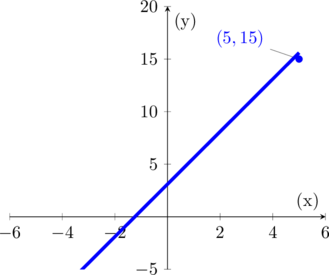# Absolute Trick for Linear Regression in ML

This is part of my notes taken while studying machine learning. I’m learning as I go along, and may update these with corrections and additional info.

In my Linear Regression for ML notes, we discussed need way to move the line to best fit our data. Two methods for doing so are:

• Absolute Trick
• Square Trick

### The Absolute Trick

The Absolute Trick is a method for moving the line closer to a point, and looks like this:

y = (w1 + p⍺)x + (w2 + ⍺)

p = the horizontal distance to the point
⍺ = (pronounced alpha) is what is referred to as the learning rate⍺,the learning rate, is a small number whose sign (+ or -) depends on if the point is above or below the line.

### Absolute Trick Example

In this example, our line is currently at y = 2x + 3 and we want to move it closer to the point at (5, 15).With a learning rate of 0.1, we are going to use the absolute trick expression:

y = 2x + 3 starting expression

y = (w1 + p⍺)x + (w2 + ⍺) move it to this

y = (2 + (5 x 0.1))x + (3 + 0.1) add our learning rate

y = 2.5x + 3.1 and now we have this.

When we plot our new expression, the line is a lot closer to our point:Next up, we have the Square Trick